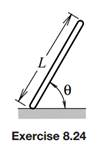### Create an Account

Home / Questions / The bar is slipping relative to the ground as it falls The coefficient of kinetic friction...

# The bar is slipping relative to the ground as it falls The coefficient of kinetic friction is µ Use Lagranges equations to derive the equations of motion for the bar

The bar is slipping relative to the ground as it falls. The coefficient of kinetic friction is µ. Use Lagrange’s equations to derive the equations of motion for the bar.Jun 16 2020 View more View LessSubscribe To Get Solution